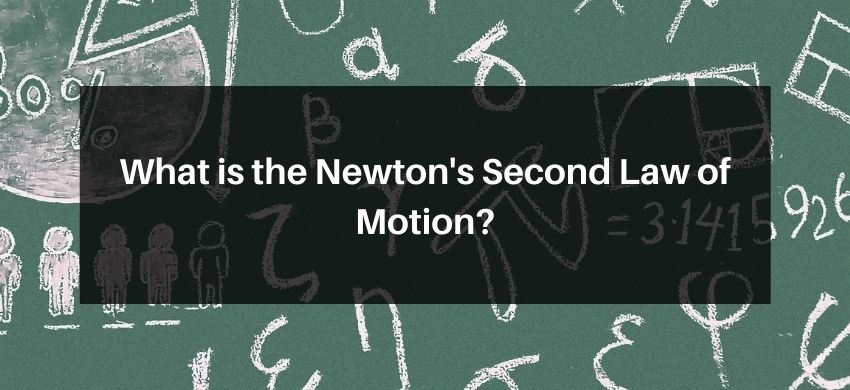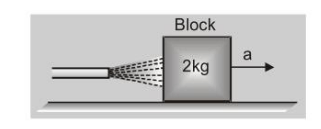Most Affordable JEE | NEET | 8,9,10 Preparation by Kota's Top IITian Doctor Faculties

# What is Newton's Second Law of Motion?## Newton's Second Law of Motion

According to this law, the rate of change of momentum is directly proportional to the applied force. The change in momentum takes place in the direction of applied force. Newton's II law gives the quantitative definition of force.

i.e. $\quad \vec{F}=\frac{\Delta \vec{p}}{\Delta t}$

If $\Delta t$ is vanishingly small then $\vec{F}=\frac{\overrightarrow{d p}}{d t}$

Out of Newton's three laws of motion, the most fundamental one is the second law, because I and III laws can be derived from it.

If external force is zero, then $\vec{F}=\frac{d \vec{p}}{d t}=0 \quad$ or $\quad \vec{p}=$ constant

or $\vec{v}=$ constant, this constant may be zero i.e. cond $^{\text {n }}$. of rest. Which is first law

APPLICATION OF NEWTON'S SECOND LAW

When force changes the state of rest or motion of a body, by Newton's Second Law

$\vec{F}=\frac{d \vec{p}}{d t}$                            .....(A)

i.e., $\vec{F}=\frac{d}{d t}(m \vec{v})$ [as $\vec{p}=m \vec{v}$ ]

i.e., $\vec{F}=m \frac{d v}{d t}+\vec{v} \frac{d m}{d t}$          ....(B)

Now for a body as $m=$ constant, $\left(\frac{d m}{d t}\right)=0$

SO      $\vec{F}=m\left(\frac{d \vec{v}}{d t}\right)$

or  $\vec{F}=m \vec{a}$ [as $\overrightarrow{\mathrm{a}}=\frac{\mathrm{d} \overrightarrow{\mathrm{v}}}{\mathrm{dt}}$ ]    ....(C)

In physics we also have situations (such as rocket motion) in which mass of the body changes

but velocity of mass is constant. In such situations as $\vec{v}=$ constant $\quad$ so $\frac{d \vec{v}}{d t}=0$,

and $e q^{n} \cdot(B)$ reduces to $\vec{F}=\vec{v} \frac{d m}{d t}$                             ....(D)

Ex. A block of metal weighing $2 \mathrm{~kg}$ is resting on a frictionless plane. It is struck by a jet releasing water at the rate of $1 \mathrm{~kg} / \mathrm{sec}$ and at a speed of $5 \mathrm{~m} / \mathrm{s}$. What will be the initial acceleration of the block?

Sol. Force exerted on block by jet of water$=\frac{\Delta}{\Delta t}(\mathrm{mv})=\frac{\Delta \mathrm{m}}{\Delta \mathrm{t}} \cdot \mathrm{v} \quad($ As $\mathrm{v}$ is constant $)$

$=(1 \mathrm{~kg} / \mathrm{sec}) \times(5 \mathrm{~m} / \mathrm{s})=5 \mathrm{~N}$

$\therefore \quad$ From relation $\mathrm{F}=$ ma

Initial acceleration of block, $\mathrm{a}=\frac{\mathrm{F}}{\mathrm{m}}=\frac{5}{2}=2.5 \mathrm{~m} / \mathrm{s}^{2}$

Newton's Second Law Provides the Definition of the Concept of Force : A force is that which causes an object to accelerate. A single force acting alone on an object has the same direction as the acceleration, and the force magnitude is proportional to the acceleration magnitude. Also, this law gives a connection between the unit of force on the one hand and the units of mass and acceleration on the other. The SI unit of force, the newton, is defined with Newton's second law:

$1 \mathrm{~N}=1 \mathrm{~kg} \cdot \mathrm{m} / \mathrm{s}^{2}$ (exactly)

Definition of the Newton (N)

If an object of mass one kilogram has an acceleration of one meter/second square relative to an inertial reference frame, then the net force exerted on the object is one newton.

CONSEQUENCES OF NEWTON'S II LAW OF MOTION

[A] Concept of inertial mass : From Newton's II law of motion $a=\frac{F}{M}$

i.e., the magnitude of acceleration produced by a given body is inversely proportional to mass i.e. greater the mass, smaller is the acceleration produced in the body. Thus, mass is the measure of inertia of the body. The mass given by above equation is therefore called the inertial mass.

[B] An accelerated motion is the result of application of the force :

There may be two types of accelerated motion:

(i) When only the magnitude of velocity of the body changes : In this types of motion the force is applied along the direction of motion or opposite to the direction of motion.

(ii) When only the direction of motion of the body changes : In this case the force is applied at right angles to the direction of motion of the body, e.g. circular motion.

[C] Acceleration produced in the body depends only on its mass and not on the final or initial velocity.